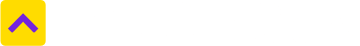Don't Miss out! Get notified of new homes as soon as they are posted

Notifications can be turned off anytime from browser settings.# Convert Decimal to Square Feet

One Decimal is equal to 435.56 Square Feet
Select State
West Bengal
Select from unit
Decimal
Select to unit
435.56
Square Feet

Real estate transactions are as mathematical in nature as they are monetary. This is why use of fractions, decimal and percentage is quite common in property sale and purchases. As for the decimal, it can be defined as a number whose integer part and the fractional part are separated by a decimal point. In other words, a decimal is applied to values with a fractional part that is separated from the integer part by the use of a decimal separator (.). The dot (.) in a decimal number is called a decimal. The digits following the decimal point show a value smaller than one.

In real estate transactions, numbers after the decimal are typically rounded off to two digits. If the decimal digit to the right of the number is rounded is 5 or higher, the digit being rounded by one. If the decimal digit to the right of the number being rounded is 4 or lower, nothing is added.

Example

5.33846 rounded to two decimals is 5.34

5.33846 rounded to one decimal is 5.3

As the percentage is more commonly used for analyzing real estate data, decimal number are often converted into percentage points. That is done by way of moving the decimal point two places to the right and replacing the decimal with a percentage sign (%).

### What are the most common land measurement units in India?

Hectares, acres, square meters and square yards are some of the common land measurement units used across India. While Bigha and Marla are commonly used in the North, terms such as Cent, Guntha and Ground belong to the South. Depending upon the names of the units, their sizes also vary from State to State.

### How much is Decimal in Square Feet terms?

One Decimal is equal to 435.56 Square Feet.

### How much is Square Feet in Decimal terms?

One Square Feet is equal to 0.00229589494 Decimal. Click here to convert
Trusted by
Partners© 2012-21 Locon Solutions Pvt. Ltd.